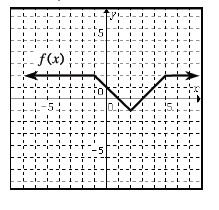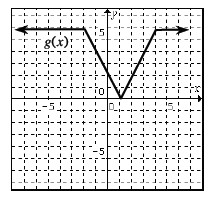### Home > PC > Chapter 3 > Lesson 3.1.3 > Problem3-44

3-44.

In the graphs below, $g\left(x\right)$ is a transformation of $f\left(x\right)$. Find an expression for $g\left(x\right)$ in terms of $f\left(x\right)$.From inspection, there is a vertical stretch by $2$, then minimum point is shifted to the left $1$ unit and a vertical shift of $2$ units.

Use the eTool below to explore these graphs.
Click on the link to the right to view the full version of the eTool: PCT 3-44 eTool